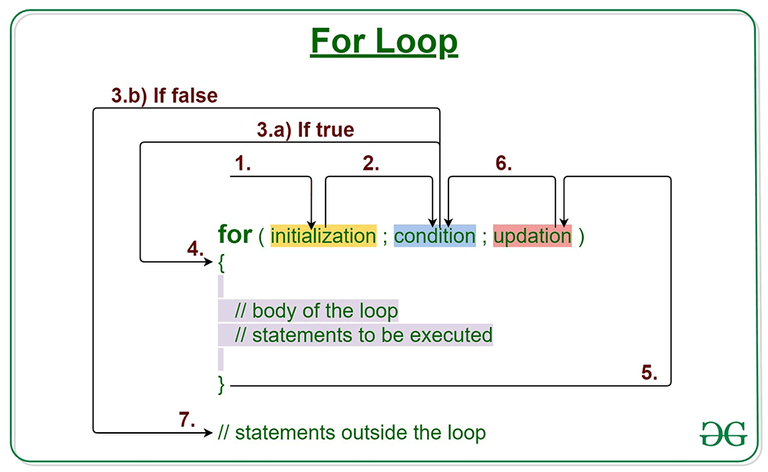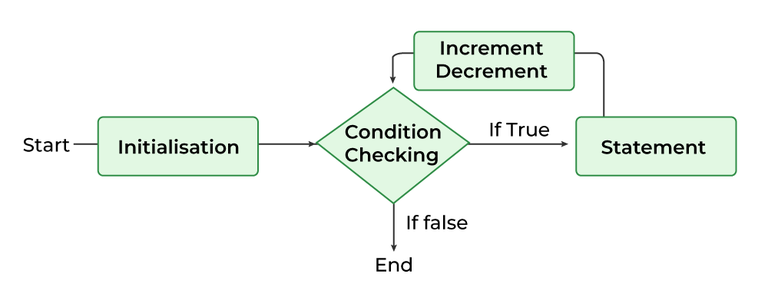GeeksforGeeks App
Open AppBrowser
Continue

## Related Articles

• Write an Interview Experience
• Java Tutorial
• Java Collection Tutorial

Loops in Java come into use when we need to repeatedly execute a block of statements. Java for loop provides a concise way of writing the loop structure. The for statement consumes the initialization, condition, and increment/decrement in one line thereby providing a shorter, easy-to-debug structure of looping. Let us understand Java for loop with Examples.Syntax:

```for (initialization expr; test expr; update exp)
{
// body of the loop
// statements we want to execute
}```

## Parts of Java For Loop

Java for loop is divided into various parts as mentioned below:

• Initialization Expression
• Test Expression
• Update Expression

### 1. Initialization Expression

In this expression, we have to initialize the loop counter to some value.

Example:

`int i=1;`

### 2. Test Expression

In this expression, we have to test the condition. If the condition evaluates to true then, we will execute the body of the loop and go to the update expression. Otherwise, we will exit from the for a loop.

Example:

`i <= 10`

### 3. Update Expression:

After executing the loop body, this expression increments/decrements the loop variable by some value.

Example:

`i++;`

## How does a For loop work?

1. Control falls into the for loop. Initialization is done
2. The flow jumps to Condition
3. Condition is tested.
• If the Condition yields true, the flow goes into the Body
• If the Condition yields false, the flow goes outside the loop
4. The statements inside the body of the loop get executed.
5. The flow goes to the Updation
6. Updation takes place and the flow goes to Step 3 again
7. The for loop has ended and the flow has gone outside.

## Flow Chart For “for loop in Java”Flow chart for loop in Java

Example 1:This program will print 1 to 10

## Java

 `/*package whatever //do not write package name here */``// Java program to write a code in for loop from 1 to 10`` ` `class` `GFG {``    ``public` `static` `void` `main(String[] args)``    ``{``        ``for` `(``int` `i = ``1``; i <= ``10``; i++) {``            ``System.out.println(i);``        ``}``    ``}``}`

Output

```1
2
3
4
5
6
7
8
9
10```

Example 2: This program will try to print “Hello World” 5 times.

## Java

 `// Java program to illustrate for loop``class` `forLoopDemo {``    ``public` `static` `void` `main(String args[])``    ``{``        ``// Writing a for loop``        ``// to print Hello World 5 times``        ``for` `(``int` `i = ``1``; i <= ``5``; i++)``            ``System.out.println(``"Hello World"``);``    ``}``}`

Output

```Hello World
Hello World
Hello World
Hello World
Hello World```

#### The complexity of the method:

```Time Complexity: O(1)
Auxiliary Space : O(1)```

### Dry-Running Example 1

The program will execute in the following manner.

1. Program starts.
2.  i is initialized with value 1.
3. Condition is checked. 1 <= 5 yields true.
1.   “Hello World” gets printed 1st time.
2.   Updation is done. Now i = 2.
4. Condition is checked. 2 <= 5 yields true.
1.   “Hello World” gets printed 2nd time.
2.   Updation is done. Now i = 3.
5. Condition is checked. 3 <= 5 yields true.
1.  “Hello World” gets printed 3rd time
2.  Updation is done. Now i = 4.
6. Condition is checked. 4 <= 5 yields true.
1. “Hello World” gets printed 4th time
2.  Updation is done. Now i = 5.
7. Condition is checked. 5 <= 5 yields true.
1.  “Hello World” gets printed 5th time
2.   Updation is done. Now i = 6.
8. Condition is checked. 6 <= 5 yields false.
9. Flow goes outside the loop. Program terminates.

Example 3: The following program prints the sum of x ranging from 1 to 20.

## Java

 `// Java program to illustrate for loop.``class` `forLoopDemo {``    ``public` `static` `void` `main(String args[])``    ``{``        ``int` `sum = ``0``;`` ` `        ``// for loop begins``        ``// and runs till x <= 20``        ``for` `(``int` `x = ``1``; x <= ``20``; x++) {``            ``sum = sum + x;``        ``}``        ``System.out.println(``"Sum: "` `+ sum);``    ``}``}`

Output

`Sum: 210`

## Java For-Each Loop

Enhanced For Loop or Java For-Each loop in Java is another version of for loop introduced in Java 5. Enhanced for loop provides a simpler way to iterate through the elements of a collection or array. It is inflexible and should be used only when there is a need to iterate through the elements in a sequential manner without knowing the index of the currently processed element.

Note: The object/variable is immutable when enhanced for loop is used i.e it ensures that the values in the array can not be modified, so it can be said as a read-only loop where you can’t update the values as opposed to other loops where values can be modified.

Syntax:

```for (T element:Collection obj/array)
{
// loop body
// statement(s)
}```

Let’s take an example to demonstrate how enhanced for loop can be used to simplify the work. Suppose there is an array of names and we want to print all the names in that array. Let’s see the difference between these two examples by this simple implementation:

## Java

 `// Java program to illustrate enhanced for loop``public` `class` `enhancedforloop {`` ` `    ``// Main Function``    ``public` `static` `void` `main(String args[])``    ``{``        ``// String array``        ``String array[] = { ``"Ron"``, ``"Harry"``, ``"Hermoine"` `};`` ` `        ``// enhanced for loop``        ``for` `(String x : array) {``            ``System.out.println(x);``        ``}`` ` `        ``/* for loop for same function``        ``for (int i = 0; i < array.length; i++)``        ``{``            ``System.out.println(array[i]);``        ``}``        ``*/``    ``}``}`

Output

```Ron
Harry
Hermoine```

Time Complexity: O(1)

Auxiliary Space : O(1)

Recommendation: Use this form of statement instead of the general form whenever possible. (as per JAVA doc.)

## Java Infinite for loop

This is an infinite loop as the condition would never return false. The initialization step is setting up the value of variable i to 1, since we are incrementing the value of i, it would always be greater than 1 so it would never return false. This would eventually lead to the infinite loop condition.

Example:

## Java

 `// Java infinite loop``class` `GFG {``    ``public` `static` `void` `main(String args[])``    ``{``        ``for` `(``int` `i = ``1``; i >= ``1``; i++) {``            ``System.out.println(``"Infinite Loop "` `+ i);``        ``}``    ``}``}`

Output:

```Infinite Loop 1
Infinite Loop 2
...```

There is another method for calling the Infinite loop

If you use two semicolons ;; in the for loop, it will be infinitive for a loop.

Syntax:

```for(;;){
//code to be executed
}  ```

Example:

## Java

 `public` `class` `GFG {`` ` `    ``public` `static` `void` `main(String[] args)``    ``{``        ``for` `(;;) {``            ``System.out.println(``"infinitive loop"``);``        ``}``    ``}``}`

Output:

```infinitive loop
infinitive loop
....```

## FAQs for Java for Loop

### 1. What is a for loop in Java?

For loop in Java is a type of loop used for the repetitive execution of a block code till the condition is fulfilled.

### 2. What is the syntax of for loop?

Syntax of for loop is mentioned below:

```for (initialization expr; test expr; update exp)
{
// body of the loop
// statements we want to execute
}```

### 3. Why is a for loop used?

For loop is used when we need to repeat the same statements for a known number of times.

Must Refer:

My Personal Notes arrow_drop_up
Related Tutorials